# CFD Simulations AC1-09

(diff) ← Older revision | Latest revision (diff) | Newer revision → (diff)

# Vortex breakdown above a delta wing with sharp leading edge

## Solution Strategy

Detached Eddy Simulations (DES) have been performed by a number of partners in the EU-project ATAAC. DES (Spalart, 2009) is a hybrid RANS–LES approach that was originally based on the Spalart–Allmaras (SA) model, but that has also been extended to ${k}$${\omega }$models. In DES switching between LES and RANS is effectively achieved by defining the turbulence length scale employed in the turbulence model as the minimum of the LES and RANS length scales. The LES length scale is the filter width, which is defined as the maximum of the mesh size in all three computational directions at each grid point. The RANS length scale depends on the RANS model employed: essentially the wall distance for the SA model and ${{\sqrt {k}}/\omega }$for the ${k}$${\omega }$model. Note that for the SA model the RANS length scale is static and therefore the RANS–LES interface is fixed, whereas for the ${k}$${\omega }$model the RANS–LES interface is dynamic.

Computations have been performed with different DES-type methods as listed in Table 1. For the underlying RANS model, the SA model (SA-DES; Spalart et al., 1997), the SST ${k}$– ${\omega }$model (SST-DES; Travin et al., 2002), and the TNT ${k}$– ${\omega }$model (X-LES; Kok et al., 2004) have been employed. The main difference between SST-DES and X-LES is that in SST-DES the blended turbulent length scale is only used to define the dissipation term in the ${k}$-equation, whereas in X-LES it is used to define the dissipation term as well as the eddy-viscosity coefficient. The delayed approach of Spalart et al. (2006), shielding attached boundary layers against inadvertently switching to LES (so-called shear-stress depletion), is used in all DES and X-LES computations (denoted as DDES and DX-LES) but one. Finally, CFSE has employed the improved variant IDDES (Shur et al., 2008). Table 1 also lists the type of solvers and grids.

Partner Model Solver Grid
National Aerospace Laboratory, Amsterdam NLR HPF DX-LES & SST-DDES ENSOLV (structured) Common & fine
Airbus Defence and Space, München CASS SST-DES TAU (unstructured) Own
German Aerospace Center, Göttingen DLR SA-DDES TAU (unstructured) Modified
Computational Fluids and Structures Engineering, Lausanne CFSE SST-IDDES NSMB (structured) Common
NUMECA International, Brussels NUM SA-DDES FINE/Open (unstructured) Common
Table 1: Models, solvers and grids used by different partners

One of the difficulties of DES consists of the development of resolved turbulence as the method switches from RANS to LES. In particular, the development of instabilities in free shear layers, such as those separating from the leading edges in the present case, may be significantly delayed. Two approaches that improve the prediction of free shear layers have been considered for X-LES: a stochastic SGS model (Kok and Van der Ven, 2002) and a high-pass-filtered (HPF) SGS model (Kok and Van der Ven, 2012). For the present case, the effect of the HPF model was significant, but the effect of the stochastic model was limited when used in combination with the HPF model. Therefore, results are mainly presented with only the HPF model. However, some of the sensitivity studies were performed with the stochastic model. Note that both approaches can be combined with other DES variants as well.

Some numerical details of the different computations are summarized in Table 2. It gives the spatial and temporal order of accuracy of the different numerical methods as well as the chosen time-step size and the time sample used to compute statistical data. The latter two are expressed in convective time units (CTU), i.e., they are made dimensionless with the free-stream velocity and the mean aerodynamic chord. NLR used a fourth-order symmetry-preserving low-dispersion finite-volume scheme with sixth-order artificial dissipation (Kok, 2009). DLR, CASS, and NUM used a standard second-order JST-type finite-volume scheme with fourth-order artificial dissipation (Jameson et al., 1981), but with a strongly reduced level of artificial dissipation. CFSE used a fourth-order central finite-volume scheme with JST-type artificial dissipation.

For time integration, all partners used the second-order backward implicit scheme. A time step of ${3.75\cdot 10^{-4}}$CTU and a mesh width of ${0.003c_{\text{mac}}}$(see grid characteristics below) imply a convective CFL number of approximately ${1/8}$based on the free-stream velocity. The experiment reports a dominant frequency of ${St=fc_{\text{mac}}/u_{\infty }=2}$implying 1,333 time steps per period. Schiavetta et al (2007) also report higher relevant frequencies, e.g., for shear layer instabilities ${St=8}$${10}$. These frequencies are also well resolved by the time step. Note that a time sample of 10 CTU implies that twenty periods of the dominant frequency have been captured.

Partner Model Space Time Grid Time step (CTU) Time sample (CTU)
NLR HPF DX-LES 4th 2nd Common 3.75×10-4 11.25
NLR HPF DX-LES 4th 2nd Fine 2.50×10-4 8.00
NLR SST-DDES 4th 2nd Common 3.75×10-4 10.50
CASS SST-DES 2nd 2nd Own 3.65×10-4 14.70
DLR SA-DDES 2nd 2nd Modified 4.00×10-4 10.00
CFSE SST-IDDES 4th 2nd Common 3.75×10-4 3.75
NUM SA-DDES 2nd 2nd Common 9.20×10-4 3.12
Table 2: Numerical methods used by different partners

## Computational Domain

The geometries of the wing and the sting are given analytically by Chu and Luckring (1996). In the computational domain, the sting is simplified: the shape of the sting upstream of the wing trailing edge conforms to the analytic definition, but it is extended as a straight cylinder from the trailing edge of the wing down to the far-field boundary (whereas the analytical definition of the sting diameter increases at some distance from the trailing edge).

NLR has generated a common multi-block structured grid, consisting of 22 blocks and 6.3 million grid cells. The grid has a conical structure over a large part of the wing: the grid covering the main vortex is essentially isotropic at each chord-wise station (outside the boundary layer) and the mesh width grows in all directions (including the stream-wise direction) together with the main vortex, going from approximately ${0.003c_{\text{mac}}}$to ${0.011c_{\text{mac}}}$. In other words, the grid resolution relative to the main vortex is kept constant. Only in a small region near the apex, the conical structure is not fully maintained, avoiding a grid singularity. The first grid cell at the wing surface has a height of ${y^{+}=O(1)}$. The far-field boundary is located at three root chord lengths from the wing in all directions, i.e., given that the apex is at the origin, the extent of the computational domain is given by ${-3c_{r}\leq x\leq 4c_{r}}$, ${-3.5c_{r}\leq y\leq 3.5c_{r}}$, and ${-3c_{r}\leq z\leq 3c_{r}}$. To study grid sensitivity, also a finer grid with the mesh width reduced by a factor 2/3 in all directions (21.4 million grid cells) as well as a modified grid with the far field located at 15 root chord lengths (8.7 million grid cells) have been generated. Additionally, CASS has generated a fine, unstructured grid (17.4 million grid cells, far field at 15 root chord lengths) that also essentially has a conical structure. An impression of the common grid is given in Figure 2.

 Figure 2: Impression of common multi-block structured grid around the delta wing (red lines are block edges)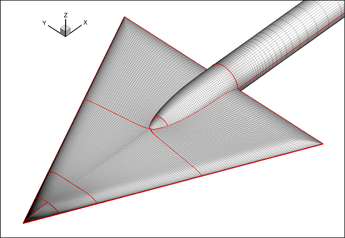a) Surface grid b) Grid in symmetry plane y = 0

## Boundary Conditions

At the wing and sting surfaces, a no-slip boundary condition is applied for the velocity and an adiabatic boundary condition is applied for the temperature. At the far field, a uniform flow is applied according the given free-stream conditions. Two far field locations have been considered, as indicated above.

## Application of Physical Models

As indicated above, DES computations are applied with either the SA model, the SST ${k}$${\omega }$model, or the TNT ${k}$${\omega }$model as underlying (RANS) turbulence model. No laminar-turbulent transition is applied, i.e., the boundary layers are assumed to be fully turbulent.

An impression of the flow is given in Figure 3, showing the instantaneous vortical structures using the Q-criterion. The main vortex above the wing is essentially captured with LES. The RANS part of the computation concerns the attached boundary layers and the near-wall region below the main vortex.

To illustrate the difficulty of capturing the instabilities developing in the free shear layers, Figure 3 shows the flow structures for a standard DES computation (SST-DDES) and for a computation with the high-pass filtered SGS model (HPF DX-LES). In the standard DES computation, the leading-edge shear layers are stable over approximately the first half of the wing (the helical structures that are visible are steady). Only towards the trailing edge, the shear layer becomes unstable and some large-scale unsteady structures are formed. In contrast, including the HPF SGS model allows the shear layer to become unstable close to the apex of the wing. Subsequently, much finer turbulent structures are formed in the shear layers and the main vortex.

 Figure 3: Instantaneous isosurface of Q = Ω2 - S2 (Q = 365 u2∞/ cr2), coloured with vorticity magnitude Ω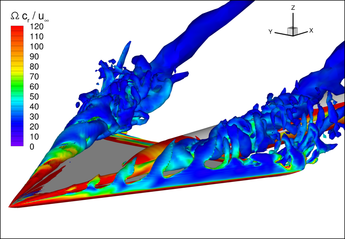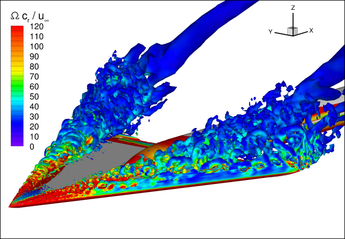a) NLR SST-DDES b) NLR HPF DX-LES

## Numerical Accuracy

For the X-LES computations of NLR, several sensitivity studies have been performed. For LES computations in general, grid convergence is a troublesome matter; the best one can do is to show the sensitivity of the result to the grid resolution. The results on the fine grid (mesh width and time step both reduced by a factor 2/3) were found to lie close to the results on the common grid, as illustrated in Figure 4 for station ${\left.x/c_{r}=0.4\right.}$(other stations show similar results). Furthermore, the sensitivity to the location of the far field, placed at three or fifteen root chord lengths from the wing, was found to be weak, as illustrated in Figure 5. Therefore, most computations have been performed with the nearest far field (smallest grid size).

 Figure 4: Solutions on common grid and fine grid of HPF DX-LES at station x/cr = 0.4 (Note: subfigures a) and b) contain the solutions on both the left side and the right side of the wing)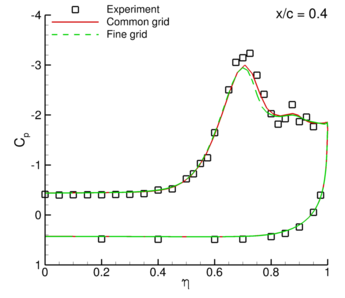a) Mean pressure coefficient b) RMS of pressure coefficient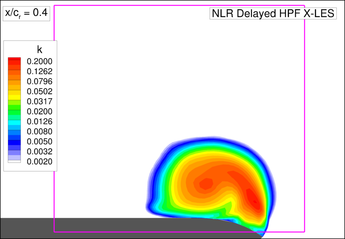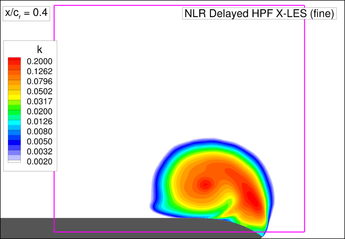c) Resolved turbulent kinetic energy ${k/u_{\infty }^{2}}$on common grid d) Resolved turbulent kinetic energy ${k/u_{\infty }^{2}}$on fine grid

 Figure 5: Solutions on common grid with different locations of far field for Stochastic HPF X-LES at station x/cr = 0.4 (Note: subfigures a) and b) contain the solutions on both the left side and the right side of the wing)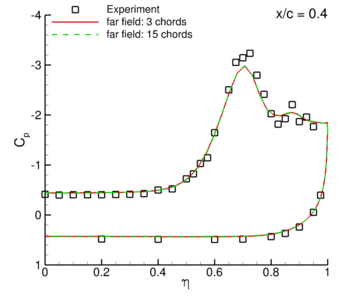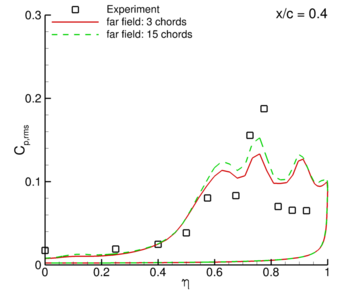a) Mean pressure coefficient b) RMS of pressure coefficient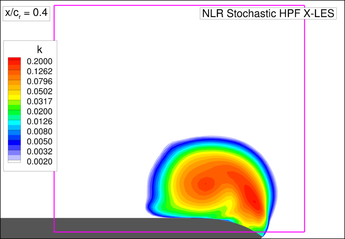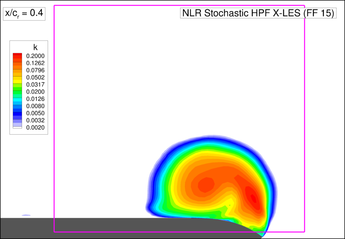c) Resolved turbulent kinetic energy ${k/u_{\infty }^{2}}$with far field at 3cr d) Resolved turbulent kinetic energy ${k/u_{\infty }^{2}}$with far field at 15cr

A strong sensitivity was found, however, to the grid point distribution. Initially, a grid had been generated with a uniform mesh width in ${x}$-direction (equal to ${0.0075c_{\text{mac}}}$), while it was conical in the other two directions. This grid was not isotropic, except in the vicinity of the expected vortex breakdown location. On this grid, a significantly lower level of resolved turbulence was obtained in the initial vortex, leading to a significantly stronger vortex and influencing the solution further downstream (Figure 6).

 Figure 6: Solutions on common grid and non-isotropic grid for Stochastic HPF X-LES at station x/cr = 0.2 (Note: subfigures a) and b) contain the solutions on both the left side and the right side of the wing)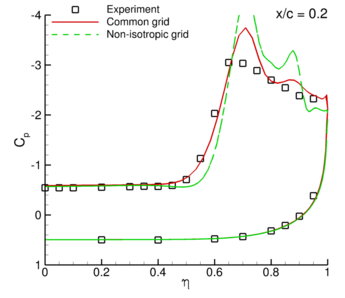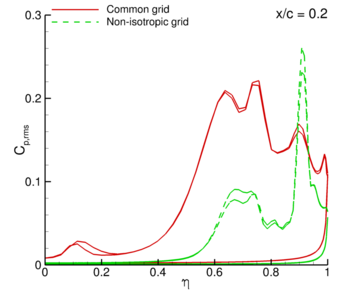a) Mean pressure coefficient b) RMS of pressure coefficient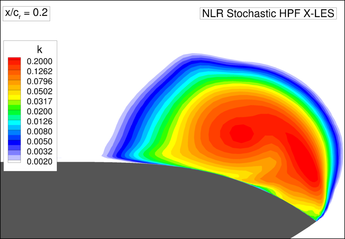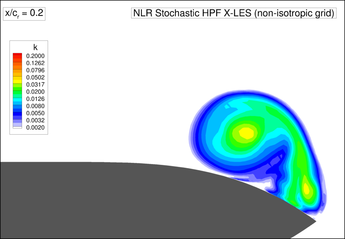c) Resolved turbulent kinetic energy ${k/u_{\infty }^{2}}$on common grid d) Resolved turbulent kinetic energy ${k/u_{\infty }^{2}}$on non-isotropic grid

Another question is how long the time sample should be to obtain statistical convergence. Using the stochastic X-LES method, a range of time samples has been considered, from 6.6 CTU to 21 CTU, see Figure 7. (For the smallest time sample, 13.2 periods of the dominant frequency are still captured.) For the mean flow quantities, statistical convergence is obtained with the smallest time sample (up to plotting accuracy). For the fluctuation quantities (in particular the RMS of ${C_{p}}$), a larger sample of about 15 CTU is needed. The smallest time sample, however, is also acceptable, because the differences with the statistically converged results are small relative to the differences found between the partners (as shown below) and the differences with the experiment. Note that the time sample should not include the transient in which the flow is still developing. The length of this transient has not been extensively investigated, but should be checked for each computation separately as it will depend on the initial solution.

 Figure 7: Solutions with varying time samples for Stochastic HPF X-LES at station x/cr = 0.4 (Note: the figures contain the solutions on both the left side and the right side of the wing)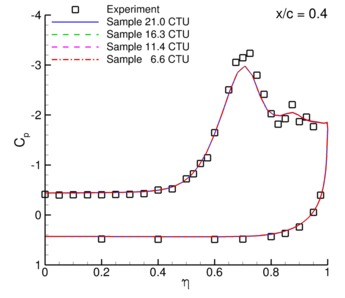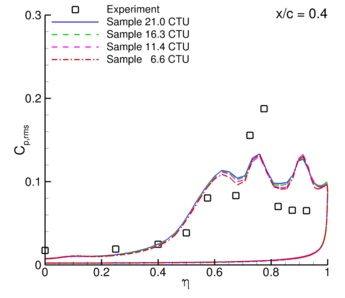a) Mean pressure coefficient b) RMS of pressure coefficient

## CFD Results

The CFD results consist of the following data:

• Time-averaged and RMS surface pressure coefficient at five chord stations:
${\left.x/c_{r}\right.=0.2}$, ${0.4}$, ${0.6}$, ${0.8}$, and ${0.95}$.
• Time-averaged velocity field and resolved turbulent kinetic energy field at five cross planes:
${\left.x/c_{r}\right.=0.2}$, ${0.4}$, ${0.6}$, ${0.8}$, and ${0.95}$.